2) Given the vectors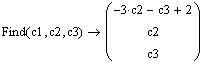Write the system equations here. The first equation is written for you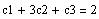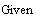<== this is to reset the values of c1,c2 and c3. Do not remove these assignments.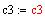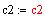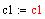Method 2 : Answer the question by using the "given ......find" command to solve the system
c1 u + c2 v + c3 w = t
For comparison, t is displayed here for you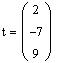==>
Step 5: Verify your answer: The is, compute c1 u + c2 v + c3 w and compare it with t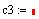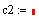Are the constants zeros? Why? ==>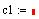b) Find the constants c1, c2 and c3 such that c1 v1 + c2 v2 + c3 v3 = 0
Step 6: Compute the row reduced echelon form of A to verify that the rank is as computed by Mathcad
Step 5: Explain why the vectors are linearly dependent or linearly independent

==>
Step 4: Click next to the arrow head and give the definition of the rank of a matrix

==>
Step 3: Use Mathcad rank() command to compute the rank of the matrix A
Step 2: Display the matrix A here ==>
Step 1: Use the augment command to form the matrix A = [ v1 v2 v3]
a) Decide if the vectors are linearly dependent or linearly independent by computing the rank of the matrix A =[v1 v2 v3], then verify the answer by computing the row reduced echelon form of A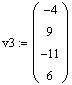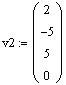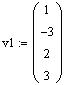==>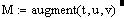Step 1 :Use the augment command to form a matrix M = [t u v w] of the vectors t, u, v and w. For example to augment the vectors t, u, and v we use
a) We know that a set of n+1 vectors in an n-dimensional vector space are linearly dependent Use the row reduced echelon form command (rref () ) in Mathcad to verify that the the vector t, u, v, and w are linearly dependent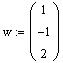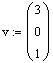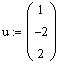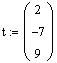1) Given the vectors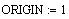Instructions:

1. Before you start working on the worksheet, make a copy of it. This can be done by saving the worksheet with a new name.
2 . To facilitate the grading of the project, type your answers after the arrow (==>) placed in every question.
3 . Do not delete any pre-assigned variables.
4. Do not add or delete lines into the document. Enough room is given for writing your answers.
5. If for some reason the screen display gets missed up, press Ctrl+R to refresh the screen display.
6 . Frequently save your worksheet to avoid the loss of your work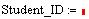Section:
List #:
Name:
Computer Project One
Math260-013 (A. Farhat)Step 4: Give the values of the constants
==>
Step 3: Compute the row reduced echelon form
==>
Step 2: Display the matrix A
==>
Step 1: Form the augmented matrix A
Method 1: Augment the vectors in a matrix A, then use the row reduce echelon form of the augmented matrix to find the constants c1, c2, c3 such that t = c1 u + c2 v +c3 w
b) Express the vector t as a linear combination of the vectors u, v and w
Explain how the row reduced echelon form is use to verify the linear dependence of the vectors